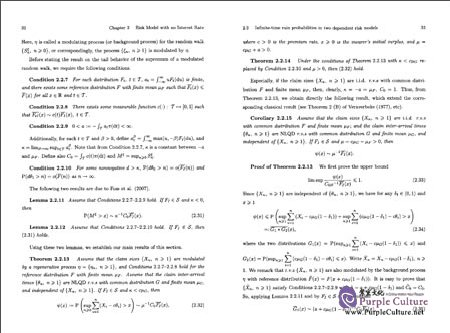FEEDBACK

#### Asymptotics and Statistical Analysis for Ruin Probabilities in Some Dependent Risk Models

Price: \$16.64 \$11.69 (Save \$4.95)
Only 1 left in stock, order soon.

• Author: Yang Yang;
• Language: English
• Format: 23.4 x 16.6 x 1 cm
• Page: 145
• Publication Date: 03/2016
• ISBN: 9787030479099, 7030479092
• Publisher: Science Press
##### Details
《相依风险模型中破产概率的渐近性与统计分析(英文版)》内容有Veraverbeke's theorem、Infinite—time ruin probabilities in two dependent risk models、Infinite—time ruin probability with modulated claim sizes、Infinite—time ruin probability with NUQD claim sizes、Finite—time ruin probabilities with NLQD inter—arrival times、Supremum of a dependent random walk with subexponential increments等。<br/>
<br/>
<b>Editor's Recommendation</b>
<p>《相依风险模型中破产概率的渐近性与统计分析(英文版)》由科学出版社出版。</p>
<br/>

《博士后文库》序言
Preface
Chapter 1 Introduction
1.1 Risk process and ruin probabilities
1.2 Claim size distributions and claim arrival process
1.2.1 Claim size and heavy—tailed or light—tailed distributions
1.2.2 The arrival process
1.3 The Cramer—Lundberg Estimate
Chapter 2 Risk Model with no Interest Rate
2.1 Veraverbeke's theorem
2.2 Infinite—time ruin probabilities in two dependent risk models
2.2.1 Infinite—time ruin probability with modulated claim sizes
2.2.2 Infinite—time ruin probability with NUQD claim sizes
2.3 Finite—time ruin probabilities with NLQD inter—arrival times
2.4 Supremum of a dependent random walk with subexponential increments
Chapter 3 Risk Model with Interest Rate
3.1 Finite—time ruin probabilities with dominatedly—varying—tailed claim sizes
3.1.1 Some existing results in the independence case
3.1.2 Asymptotics and uniform asymptotics for finite—time ruin probabilities in the dependence case
3.2 Further results on asymptotics and uniform asymptotics for ruin probabilities with dominatedly—varying—tailed claim sizes
3.3 Finite—time ruin probabilities with subexponential claim sizes in the dependent compound renewal risk model
3.3.1 Compound renewal risk model and dependence structures
3.3.2 Finite—time ruin probabilities with subexponentialindividual claim sizes
3.3.3 Simulation study
Chapter 4 Discrete—time Risk Model with Insurance and Financial Risks
4.1 Randomly weighted sums in the independence case
4.1.1 Randomly weighted finite sums
4.1.2 Randomly weighted infinite sums
4.2 Randomly weighted sums in the dependence case
4.2.1 Randomly weighted finite sums with dependent primary random variables
4.2.2 Randomly weighted finite sums with dependence between the primary random variable and the random weight
4.2.3 Randomly weighted infinite sums with dependent primary random variables
Bibliography

##### Sample Pages Preview\$11.69